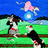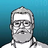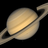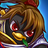V2EX = way to explore
V2EX 是一个关于分享和探索的地方

V2EX  ›  C++

# 5 分钟掌握 C++中的三种继承方式

yiouejv · 276 天前 · 1944 次点击

### public 方式继承

``````class A
{
private:
int m_data1;
void print1() { cout << "private print1" << endl; }
protected:
int m_data2;
void print2() { cout << "protected print2" << endl; }
public:
A(int x = 1, int y = 2, int z = 3) : m_data1(x), m_data2(y), m_data3(z) {}
int m_data3;
void print3() { cout << "protected print3" << endl; }
};

class B : public A
{
public:
void test_public() {
cout << m_data3 << endl;
print3();
}
void test_protected() {
cout << m_data2 << endl;
print2();
}
void test_private() {
// 下面两行编译不过，B 类内无法访问父类的私有成员
// cout << m_data1 << endl;
// print1();
}
};

int main(int argc, char const* argv[])
{
B b;
b.test_public();
b.test_protected();
b.test_private();
cout << b.m_data3 << endl;
// cout << b.m_data2 << endl;  // 编译不过，子类对象无法访问父类 protected 的成员
// cout << b.m_data1 << endl;  // 编译不过，子类对象无法访问父类 private 的成员
return 0;
}
``````

### private 方式继承

``````class A
{
private:
int m_data1;
void print1() { cout << "private print1" << endl; }
protected:
int m_data2;
void print2() { cout << "protected print2" << endl; }
public:
A(int x = 1, int y = 2, int z = 3) : m_data1(x), m_data2(y), m_data3(z) {}
int m_data3;
void print3() { cout << "protected print3" << endl; }
};

class B : private A
{
public:
void test_public() {
cout << m_data3 << endl;
print3();
}
void test_protected() {
cout << m_data2 << endl;
print2();
}
void test_private() {
// 下面两行编译不过，B 类内无法访问父类的私有成员
// cout << m_data1 << endl;
// print1();
}
};

int main(int argc, char const* argv[])
{
B b;
b.test_public();
b.test_protected();
b.test_private();
// cout << b.m_data3 << endl;  // // 编译不过，子类对象无法访问父类 public 的成员
// cout << b.m_data2 << endl;  // 编译不过，子类对象无法访问父类 protected 的成员
// cout << b.m_data1 << endl;  // 编译不过，子类对象无法访问父类 private 的成员
return 0;
}
``````

### protected 方式继承

public 的方式继承到派生类，这些成员的权限和在基类里的权限保持一致;
protected 方式继承到派生类，成员的权限都变为 protected;
private 方式继承到派生类，成员的权限都变为 private;

``````#include<iostream>
#include<cstdio>

class Parent
{
public:
Parent(int var=-1) {
m_nPub = var;
m_nPtd = var;
m_bPrt = var;
}
int m_nPub;
protected:
int m_nPtd;
private:
int m_nPrt;
};

class Child1 : public Parent
{
public:
int GetPub() { return m_nPub; }
int GetPtd() { return m_nPtd; }
int GetPrt() { return m_nPrt; }
// A
};

class Child2 : protected Parent
{
public:
int GetPub() { return m_nPub; }
int GetPtd() { return m_nPtd; }
int GetPrt() { return m_nPrt; }
// B
};

class Child3 : private Parent
{
public:
int GetPub() { return m_nPub; }
int GetPtd() { return m_nPtd; }
int GetPrt() { return m_nPrt; }
// C
};

int main(int argc, char const *argv[])
{
Child1 cd1;
Child2 cd2;
Child3 cd3;

int nVar = 0;

// public inherited
cd1.m_nPub = nVar; // D
cd1.m_nPtd = nVar; // E
nVar = cd1.GetPtd(); // F

// protected inherited
cd2.m_nPub = nVar; // G
nVar = cd2.GetPtd(); // H

// private inherited
cd3.m_nPub = nVar; // I
nVar = cd3.GetPtd();  // J
return 0;
}
``````

A, B, C 都错误，因为 m_nPrt 是父类的 private 变量，子类不能访问。

D 正确。cdl 是公有继承，可以访问并改变父类的公有变量。

E 错误。m_nPtd 是父类 Parent 的保护变量，不可以被公有继承的 cdl 访问， 更不可以被修改。 虽然 m_nPtd 是父类 Parent 的保护变量，经过公有继承后，m_nPtd 在子类中依然是 protected, 而子类的对象 cdl 是不能访问自身的 protected 成员，只能访问 public 成员。

F 正确。派生类内可以访问父类的保护变量。

G 错误。cd2 是保护继承的，派生类对象不能访问父类成员。

H 正确。派生类内可以访问父类的保护变量。

I 错误。cd2 是私有继承的，派生类对象不能访问父类成员。

J 正确。派生类内可以访问父类的保护变量。8 条回复    2021-03-01 23:16:23 +08:001 xiaoxinxiaobai   276 天前 via Android 看完你这点东西都不止 5 分钟2 auto8888   276 天前 派生类都可以访问基类的 public, protected 成员。public：继承过来权限不变，派生类实例化的对象只能访问基类 public 成员。private：继承过来之后变成自己私有的， 派生类实例化的对象啥都不能访问。protected：继承过来公有成员和保护成员都变成了 protected，派生类实例化的对象啥都不能访问。总结了下，5 分钟读完。。。3 yiouejv   276 天前 @xiaoxinxiaobai 只看总结 1 分钟就学会了，手动狗头4 LigeLaige   276 天前 可以再介绍一下，它们有哪些经典的用法，使用场景举例。5 dangyuluo   276 天前 为什么学什么都需要速成6 Lemeng   276 天前 总之，绑定一下7 smileli   274 天前 支持一波8 yiouejv   274 天前 @smileli 关注一波